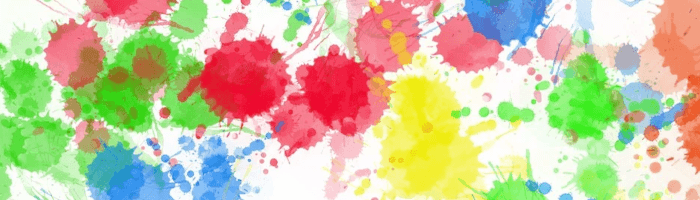# Image Deduplicator – find duplicate images

### In Operation

Here’s a very simple example of using Image Deduplicator.

Let’s create a Python script (named test.py). The script checks the /home/sde/Pics directory, and looks for identical or near-identical images to the moving-forward-linux.png image.

Our script contains the following lines. It’s using the perceptual hashing method.

`from imagededup.methods import PHash`
`phasher = PHash()`
`encodings = phasher.encode_images(image_dir='/home/sde/Pics')`
`duplicates = phasher.find_duplicates(encoding_map=encodings)`
`from imagededup.utils import plot_duplicates`
`plot_duplicates(image_dir='/home/sde/Pics', duplicate_map=duplicates, filename='moving-forward-linux.png')`

Running the Python script (with ` python test.py` generates the following output.This shows that we have 3 identical or near-identical duplicate images.

In the example, we used the perceptual hashing algorithm. Image Deduplicator offers a variety of hashing algorithms to find exact duplicates and near duplicates.

• Convolutional Neural Network (CNN) – a Deep Learning algorithm which can take in an input image, assign importance (learnable weights and biases) to various aspects/objects in the image and be able to differentiate one from the other.
• Perceptual hashing (PHash) – describes a class of comparable hash functions. A perceptual hash is like a regular hash in that it is a smaller, comparable fingerprint of a much larger piece of data. However, unlike a typical hashing algorithm, the idea with a perceptual hash is that the perceptual hashes are “close” (or equal) if the original images are close. They are used for multimedia content identification and authentication through perception digests based on the understanding of multimedia content.
• Difference hashing (DHash) – this is a perceptual image hash which provides a simple but very effective algorithm.
• Wavelet hashing (WHash) – works in the frequency domain as PHash but it uses Discrete Wavelet Transform (DWT) instead of Discrete Cosine Transform (DCT).
• Average hashing (AHash) – average hash, for each of the pixels output 1 if the pixel is bigger or equal to the average and 0 otherwise.

Next page: Page 3 – Summary